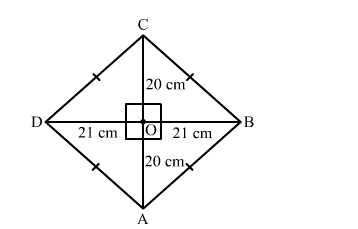# The length of the diagonals of a rhombus are 40 cm and 42 cm.Question:

The length of the diagonals of a rhombus are 40 cm and 42 cm. Find the length of each sides of the rhombus

Solution:Suppose ABCD is a rhombus.
We know that the digonals of a rhombus perpendicularly bisect each other.
∴ ∠AOB = 900, AO = 20 cm and BO = 21 cm
Now, In right triangle AOB
By using Pythagoras theorem we have
AB2 = AO2 + OB2
= 202 + 212
= 400 + 441
= 841
∴AB2 = 841
⇒ AB = 29 cm
Since, all the sides of a rhombus are equal.
Hence, AB = BC = CD = DA = 29 cm